Open in App
Not now

# Merge Sort Algorithm

• Difficulty Level : Medium
• Last Updated : 17 Feb, 2023

Merge sort is a sorting algorithm that works by dividing an array into smaller subarrays, sorting each subarray, and then merging the sorted subarrays back together to form the final sorted array.

In simple terms, we can say that the process of merge sort is to divide the array into two halves, sort each half, and then merge the sorted halves back together. This process is repeated until the entire array is sorted.

One thing that you might wonder is what is the specialty of this algorithm. We already have a number of sorting algorithms then why do we need this algorithm? One of the main advantages of merge sort is that it has a time complexity of O(n log n), which means it can sort large arrays relatively quickly. It is also a stable sort, which means that the order of elements with equal values is preserved during the sort.

Merge sort is a popular choice for sorting large datasets because it is relatively efficient and easy to implement. It is often used in conjunction with other algorithms, such as quicksort, to improve the overall performance of a sorting routine.

## Merge Sort Working Process:

Think of it as a recursive algorithm continuously splits the array in half until it cannot be further divided. This means that if the array becomes empty or has only one element left, the dividing will stop, i.e. it is the base case to stop the recursion. If the array has multiple elements, split the array into halves and recursively invoke the merge sort on each of the halves. Finally, when both halves are sorted, the merge operation is applied. Merge operation is the process of taking two smaller sorted arrays and combining them to eventually make a larger one.

## Illustration:

To know the functioning of merge sort, lets consider an array arr[] = {38, 27, 43, 3, 9, 82, 10}

• At first, check if the left index of array is less than the right index, if yes then calculate its mid point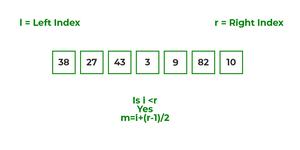• Now, as we already know that merge sort first divides the whole array iteratively into equal halves, unless the atomic values are achieved.
• Here, we see that an array of 7 items is divided into two arrays of size 4 and 3 respectively.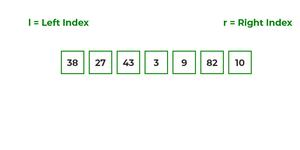• Now, again find that is left index is less than the right index for both arrays, if found yes, then again calculate mid points for both the arrays.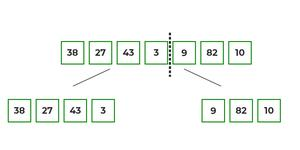• Now, further divide these two arrays into further halves, until the atomic units of the array is reached and further division is not possible.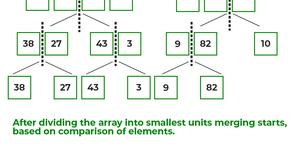• After dividing the array into smallest units, start merging the elements again based on comparison of size of elements
• Firstly, compare the element for each list and then combine them into another list in a sorted manner.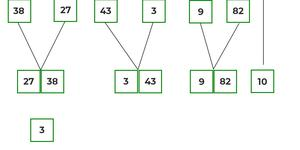• After the final merging, the list looks like this: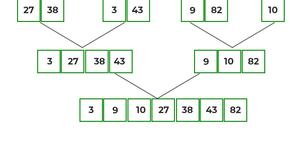The following diagram shows the complete merge sort process for an example array {38, 27, 43, 3, 9, 82, 10}.

If we take a closer look at the diagram, we can see that the array is recursively divided into two halves till the size becomes 1. Once the size becomes 1, the merge processes come into action and start merging arrays back till the complete array is merged.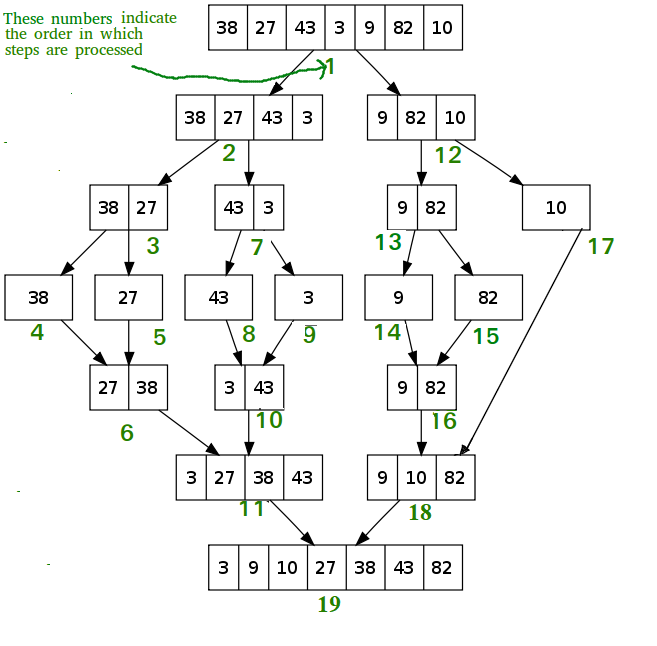Recursive steps of merge sort

Recommended Practice

## Algorithm:

step 1: start

step 2: declare array and left, right, mid variable

step 3: perform merge function.
if left > right
return
mid= (left+right)/2
mergesort(array, left, mid)
mergesort(array, mid+1, right)
merge(array, left, mid, right)

step 4: Stop

Follow the steps below to solve the problem:

MergeSort(arr[], l,  r)
If r > l

• Find the middle point to divide the array into two halves:
• middle m = l + (r – l)/2
• Call mergeSort for first half:
• Call mergeSort(arr, l, m)
• Call mergeSort for second half:
• Call mergeSort(arr, m + 1, r)
• Merge the two halves sorted in steps 2 and 3:
• Call merge(arr, l, m, r)

Below is the implementation of the above approach:

## C++

 `// C++ program for Merge Sort ` `#include ` `using` `namespace` `std; ` ` `  `// Merges two subarrays of array[]. ` `// First subarray is arr[begin..mid] ` `// Second subarray is arr[mid+1..end] ` `void` `merge(``int` `array[], ``int` `const` `left, ``int` `const` `mid, ` `           ``int` `const` `right) ` `{ ` `    ``auto` `const` `subArrayOne = mid - left + 1; ` `    ``auto` `const` `subArrayTwo = right - mid; ` ` `  `    ``// Create temp arrays ` `    ``auto` `*leftArray = ``new` `int``[subArrayOne], ` `         ``*rightArray = ``new` `int``[subArrayTwo]; ` ` `  `    ``// Copy data to temp arrays leftArray[] and rightArray[] ` `    ``for` `(``auto` `i = 0; i < subArrayOne; i++) ` `        ``leftArray[i] = array[left + i]; ` `    ``for` `(``auto` `j = 0; j < subArrayTwo; j++) ` `        ``rightArray[j] = array[mid + 1 + j]; ` ` `  `    ``auto` `indexOfSubArrayOne ` `        ``= 0, ``// Initial index of first sub-array ` `        ``indexOfSubArrayTwo ` `        ``= 0; ``// Initial index of second sub-array ` `    ``int` `indexOfMergedArray ` `        ``= left; ``// Initial index of merged array ` ` `  `    ``// Merge the temp arrays back into array[left..right] ` `    ``while` `(indexOfSubArrayOne < subArrayOne ` `           ``&& indexOfSubArrayTwo < subArrayTwo) { ` `        ``if` `(leftArray[indexOfSubArrayOne] ` `            ``<= rightArray[indexOfSubArrayTwo]) { ` `            ``array[indexOfMergedArray] ` `                ``= leftArray[indexOfSubArrayOne]; ` `            ``indexOfSubArrayOne++; ` `        ``} ` `        ``else` `{ ` `            ``array[indexOfMergedArray] ` `                ``= rightArray[indexOfSubArrayTwo]; ` `            ``indexOfSubArrayTwo++; ` `        ``} ` `        ``indexOfMergedArray++; ` `    ``} ` `    ``// Copy the remaining elements of ` `    ``// left[], if there are any ` `    ``while` `(indexOfSubArrayOne < subArrayOne) { ` `        ``array[indexOfMergedArray] ` `            ``= leftArray[indexOfSubArrayOne]; ` `        ``indexOfSubArrayOne++; ` `        ``indexOfMergedArray++; ` `    ``} ` `    ``// Copy the remaining elements of ` `    ``// right[], if there are any ` `    ``while` `(indexOfSubArrayTwo < subArrayTwo) { ` `        ``array[indexOfMergedArray] ` `            ``= rightArray[indexOfSubArrayTwo]; ` `        ``indexOfSubArrayTwo++; ` `        ``indexOfMergedArray++; ` `    ``} ` `    ``delete``[] leftArray; ` `    ``delete``[] rightArray; ` `} ` ` `  `// begin is for left index and end is ` `// right index of the sub-array ` `// of arr to be sorted */ ` `void` `mergeSort(``int` `array[], ``int` `const` `begin, ``int` `const` `end) ` `{ ` `    ``if` `(begin >= end) ` `        ``return``; ``// Returns recursively ` ` `  `    ``auto` `mid = begin + (end - begin) / 2; ` `    ``mergeSort(array, begin, mid); ` `    ``mergeSort(array, mid + 1, end); ` `    ``merge(array, begin, mid, end); ` `} ` ` `  `// UTILITY FUNCTIONS ` `// Function to print an array ` `void` `printArray(``int` `A[], ``int` `size) ` `{ ` `    ``for` `(``auto` `i = 0; i < size; i++) ` `        ``cout << A[i] << ``" "``; ` `} ` ` `  `// Driver code ` `int` `main() ` `{ ` `    ``int` `arr[] = { 12, 11, 13, 5, 6, 7 }; ` `    ``auto` `arr_size = ``sizeof``(arr) / ``sizeof``(arr); ` ` `  `    ``cout << ``"Given array is \n"``; ` `    ``printArray(arr, arr_size); ` ` `  `    ``mergeSort(arr, 0, arr_size - 1); ` ` `  `    ``cout << ``"\nSorted array is \n"``; ` `    ``printArray(arr, arr_size); ` `    ``return` `0; ` `} ` ` `  `// This code is contributed by Mayank Tyagi ` `// This code was revised by Joshua Estes`

## C

 `/* C program for Merge Sort */` `#include ` `#include ` ` `  `// Merges two subarrays of arr[]. ` `// First subarray is arr[l..m] ` `// Second subarray is arr[m+1..r] ` `void` `merge(``int` `arr[], ``int` `l, ``int` `m, ``int` `r) ` `{ ` `    ``int` `i, j, k; ` `    ``int` `n1 = m - l + 1; ` `    ``int` `n2 = r - m; ` ` `  `    ``/* create temp arrays */` `    ``int` `L[n1], R[n2]; ` ` `  `    ``/* Copy data to temp arrays L[] and R[] */` `    ``for` `(i = 0; i < n1; i++) ` `        ``L[i] = arr[l + i]; ` `    ``for` `(j = 0; j < n2; j++) ` `        ``R[j] = arr[m + 1 + j]; ` ` `  `    ``/* Merge the temp arrays back into arr[l..r]*/` `    ``i = 0; ``// Initial index of first subarray ` `    ``j = 0; ``// Initial index of second subarray ` `    ``k = l; ``// Initial index of merged subarray ` `    ``while` `(i < n1 && j < n2) { ` `        ``if` `(L[i] <= R[j]) { ` `            ``arr[k] = L[i]; ` `            ``i++; ` `        ``} ` `        ``else` `{ ` `            ``arr[k] = R[j]; ` `            ``j++; ` `        ``} ` `        ``k++; ` `    ``} ` ` `  `    ``/* Copy the remaining elements of L[], if there ` `    ``are any */` `    ``while` `(i < n1) { ` `        ``arr[k] = L[i]; ` `        ``i++; ` `        ``k++; ` `    ``} ` ` `  `    ``/* Copy the remaining elements of R[], if there ` `    ``are any */` `    ``while` `(j < n2) { ` `        ``arr[k] = R[j]; ` `        ``j++; ` `        ``k++; ` `    ``} ` `} ` ` `  `/* l is for left index and r is right index of the ` `sub-array of arr to be sorted */` `void` `mergeSort(``int` `arr[], ``int` `l, ``int` `r) ` `{ ` `    ``if` `(l < r) { ` `        ``// Same as (l+r)/2, but avoids overflow for ` `        ``// large l and h ` `        ``int` `m = l + (r - l) / 2; ` ` `  `        ``// Sort first and second halves ` `        ``mergeSort(arr, l, m); ` `        ``mergeSort(arr, m + 1, r); ` ` `  `        ``merge(arr, l, m, r); ` `    ``} ` `} ` ` `  `/* UTILITY FUNCTIONS */` `/* Function to print an array */` `void` `printArray(``int` `A[], ``int` `size) ` `{ ` `    ``int` `i; ` `    ``for` `(i = 0; i < size; i++) ` `        ``printf``(``"%d "``, A[i]); ` `    ``printf``(``"\n"``); ` `} ` ` `  `/* Driver code */` `int` `main() ` `{ ` `    ``int` `arr[] = { 12, 11, 13, 5, 6, 7 }; ` `    ``int` `arr_size = ``sizeof``(arr) / ``sizeof``(arr); ` ` `  `    ``printf``(``"Given array is \n"``); ` `    ``printArray(arr, arr_size); ` ` `  `    ``mergeSort(arr, 0, arr_size - 1); ` ` `  `    ``printf``(``"\nSorted array is \n"``); ` `    ``printArray(arr, arr_size); ` `    ``return` `0; ` `}`

## Java

 `/* Java program for Merge Sort */` `class` `MergeSort { ` `    ``// Merges two subarrays of arr[]. ` `    ``// First subarray is arr[l..m] ` `    ``// Second subarray is arr[m+1..r] ` `    ``void` `merge(``int` `arr[], ``int` `l, ``int` `m, ``int` `r) ` `    ``{ ` `        ``// Find sizes of two subarrays to be merged ` `        ``int` `n1 = m - l + ``1``; ` `        ``int` `n2 = r - m; ` ` `  `        ``/* Create temp arrays */` `        ``int` `L[] = ``new` `int``[n1]; ` `        ``int` `R[] = ``new` `int``[n2]; ` ` `  `        ``/*Copy data to temp arrays*/` `        ``for` `(``int` `i = ``0``; i < n1; ++i) ` `            ``L[i] = arr[l + i]; ` `        ``for` `(``int` `j = ``0``; j < n2; ++j) ` `            ``R[j] = arr[m + ``1` `+ j]; ` ` `  `        ``/* Merge the temp arrays */` ` `  `        ``// Initial indexes of first and second subarrays ` `        ``int` `i = ``0``, j = ``0``; ` ` `  `        ``// Initial index of merged subarray array ` `        ``int` `k = l; ` `        ``while` `(i < n1 && j < n2) { ` `            ``if` `(L[i] <= R[j]) { ` `                ``arr[k] = L[i]; ` `                ``i++; ` `            ``} ` `            ``else` `{ ` `                ``arr[k] = R[j]; ` `                ``j++; ` `            ``} ` `            ``k++; ` `        ``} ` ` `  `        ``/* Copy remaining elements of L[] if any */` `        ``while` `(i < n1) { ` `            ``arr[k] = L[i]; ` `            ``i++; ` `            ``k++; ` `        ``} ` ` `  `        ``/* Copy remaining elements of R[] if any */` `        ``while` `(j < n2) { ` `            ``arr[k] = R[j]; ` `            ``j++; ` `            ``k++; ` `        ``} ` `    ``} ` ` `  `    ``// Main function that sorts arr[l..r] using ` `    ``// merge() ` `    ``void` `sort(``int` `arr[], ``int` `l, ``int` `r) ` `    ``{ ` `        ``if` `(l < r) { ` `            ``// Find the middle point ` `            ``int` `m = l + (r - l) / ``2``; ` ` `  `            ``// Sort first and second halves ` `            ``sort(arr, l, m); ` `            ``sort(arr, m + ``1``, r); ` ` `  `            ``// Merge the sorted halves ` `            ``merge(arr, l, m, r); ` `        ``} ` `    ``} ` ` `  `    ``/* A utility function to print array of size n */` `    ``static` `void` `printArray(``int` `arr[]) ` `    ``{ ` `        ``int` `n = arr.length; ` `        ``for` `(``int` `i = ``0``; i < n; ++i) ` `            ``System.out.print(arr[i] + ``" "``); ` `        ``System.out.println(); ` `    ``} ` ` `  `    ``// Driver code ` `    ``public` `static` `void` `main(String args[]) ` `    ``{ ` `        ``int` `arr[] = { ``12``, ``11``, ``13``, ``5``, ``6``, ``7` `}; ` ` `  `        ``System.out.println(``"Given Array"``); ` `        ``printArray(arr); ` ` `  `        ``MergeSort ob = ``new` `MergeSort(); ` `        ``ob.sort(arr, ``0``, arr.length - ``1``); ` ` `  `        ``System.out.println(``"\nSorted array"``); ` `        ``printArray(arr); ` `    ``} ` `} ` `/* This code is contributed by Rajat Mishra */`

## Python3

 `# Python program for implementation of MergeSort ` `def` `mergeSort(arr): ` `    ``if` `len``(arr) > ``1``: ` ` `  `         ``# Finding the mid of the array ` `        ``mid ``=` `len``(arr)``/``/``2` ` `  `        ``# Dividing the array elements ` `        ``L ``=` `arr[:mid] ` ` `  `        ``# into 2 halves ` `        ``R ``=` `arr[mid:] ` ` `  `        ``# Sorting the first half ` `        ``mergeSort(L) ` ` `  `        ``# Sorting the second half ` `        ``mergeSort(R) ` ` `  `        ``i ``=` `j ``=` `k ``=` `0` ` `  `        ``# Copy data to temp arrays L[] and R[] ` `        ``while` `i < ``len``(L) ``and` `j < ``len``(R): ` `            ``if` `L[i] <``=` `R[j]: ` `                ``arr[k] ``=` `L[i] ` `                ``i ``+``=` `1` `            ``else``: ` `                ``arr[k] ``=` `R[j] ` `                ``j ``+``=` `1` `            ``k ``+``=` `1` ` `  `        ``# Checking if any element was left ` `        ``while` `i < ``len``(L): ` `            ``arr[k] ``=` `L[i] ` `            ``i ``+``=` `1` `            ``k ``+``=` `1` ` `  `        ``while` `j < ``len``(R): ` `            ``arr[k] ``=` `R[j] ` `            ``j ``+``=` `1` `            ``k ``+``=` `1` ` `  `# Code to print the list ` ` `  ` `  `def` `printList(arr): ` `    ``for` `i ``in` `range``(``len``(arr)): ` `        ``print``(arr[i], end``=``" "``) ` `    ``print``() ` ` `  ` `  `# Driver Code ` `if` `__name__ ``=``=` `'__main__'``: ` `    ``arr ``=` `[``12``, ``11``, ``13``, ``5``, ``6``, ``7``] ` `    ``print``(``"Given array is"``, end``=``"\n"``) ` `    ``printList(arr) ` `    ``mergeSort(arr) ` `    ``print``(``"Sorted array is: "``, end``=``"\n"``) ` `    ``printList(arr) ` ` `  `# This code is contributed by Mayank Khanna `

## C#

 `// C# program for Merge Sort ` `using` `System; ` `class` `MergeSort { ` ` `  `    ``// Merges two subarrays of []arr. ` `    ``// First subarray is arr[l..m] ` `    ``// Second subarray is arr[m+1..r] ` `    ``void` `merge(``int``[] arr, ``int` `l, ``int` `m, ``int` `r) ` `    ``{ ` `        ``// Find sizes of two ` `        ``// subarrays to be merged ` `        ``int` `n1 = m - l + 1; ` `        ``int` `n2 = r - m; ` ` `  `        ``// Create temp arrays ` `        ``int``[] L = ``new` `int``[n1]; ` `        ``int``[] R = ``new` `int``[n2]; ` `        ``int` `i, j; ` ` `  `        ``// Copy data to temp arrays ` `        ``for` `(i = 0; i < n1; ++i) ` `            ``L[i] = arr[l + i]; ` `        ``for` `(j = 0; j < n2; ++j) ` `            ``R[j] = arr[m + 1 + j]; ` ` `  `        ``// Merge the temp arrays ` ` `  `        ``// Initial indexes of first ` `        ``// and second subarrays ` `        ``i = 0; ` `        ``j = 0; ` ` `  `        ``// Initial index of merged ` `        ``// subarray array ` `        ``int` `k = l; ` `        ``while` `(i < n1 && j < n2) { ` `            ``if` `(L[i] <= R[j]) { ` `                ``arr[k] = L[i]; ` `                ``i++; ` `            ``} ` `            ``else` `{ ` `                ``arr[k] = R[j]; ` `                ``j++; ` `            ``} ` `            ``k++; ` `        ``} ` ` `  `        ``// Copy remaining elements ` `        ``// of L[] if any ` `        ``while` `(i < n1) { ` `            ``arr[k] = L[i]; ` `            ``i++; ` `            ``k++; ` `        ``} ` ` `  `        ``// Copy remaining elements ` `        ``// of R[] if any ` `        ``while` `(j < n2) { ` `            ``arr[k] = R[j]; ` `            ``j++; ` `            ``k++; ` `        ``} ` `    ``} ` ` `  `    ``// Main function that ` `    ``// sorts arr[l..r] using ` `    ``// merge() ` `    ``void` `sort(``int``[] arr, ``int` `l, ``int` `r) ` `    ``{ ` `        ``if` `(l < r) { ` `            ``// Find the middle ` `            ``// point ` `            ``int` `m = l + (r - l) / 2; ` ` `  `            ``// Sort first and ` `            ``// second halves ` `            ``sort(arr, l, m); ` `            ``sort(arr, m + 1, r); ` ` `  `            ``// Merge the sorted halves ` `            ``merge(arr, l, m, r); ` `        ``} ` `    ``} ` ` `  `    ``// A utility function to ` `    ``// print array of size n */ ` `    ``static` `void` `printArray(``int``[] arr) ` `    ``{ ` `        ``int` `n = arr.Length; ` `        ``for` `(``int` `i = 0; i < n; ++i) ` `            ``Console.Write(arr[i] + ``" "``); ` `        ``Console.WriteLine(); ` `    ``} ` ` `  `    ``// Driver code ` `    ``public` `static` `void` `Main(String[] args) ` `    ``{ ` `        ``int``[] arr = { 12, 11, 13, 5, 6, 7 }; ` `        ``Console.WriteLine(``"Given Array"``); ` `        ``printArray(arr); ` `        ``MergeSort ob = ``new` `MergeSort(); ` `        ``ob.sort(arr, 0, arr.Length - 1); ` `        ``Console.WriteLine(``"\nSorted array"``); ` `        ``printArray(arr); ` `    ``} ` `} ` ` `  `// This code is contributed by Princi Singh`

## Javascript

 ` `

## PHP

 ` `

Output

```Given array is
12 11 13 5 6 7
Sorted array is
5 6 7 11 12 13 ```

Time Complexity: O(N log(N)),  Sorting arrays on different machines. Merge Sort is a recursive algorithm and time complexity can be expressed as following recurrence relation.

T(n) = 2T(n/2) + θ(n)

The above recurrence can be solved either using the Recurrence Tree method or the Master method. It falls in case II of the Master Method and the solution of the recurrence is θ(Nlog(N)). The time complexity of Merge Sort isθ(Nlog(N)) in all 3 cases (worst, average, and best) as merge sort always divides the array into two halves and takes linear time to merge two halves.

Auxiliary Space: O(n), In merge sort all elements are copied into an auxiliary array. So N auxiliary space is required for merge sort.

## Is Merge sort In Place?

No, In merge sort the merging step requires extra space to store the elements.

## Is Merge sort Stable?

Yes, merge sort is stable.

## How can we make Merge sort more efficient?

Merge sort can be made more efficient by replacing recursive calls with Insertion sort for smaller array sizes, where the size of the remaining array is less or equal to 43 as the number of operations required to sort an array of max size 43 will be less in Insertion sort as compared to the number of operations required in Merge sort.

## Analysis of Merge Sort:

A merge sort consists of several passes over the input. The first pass merges segments of size 1, the second merges segments of size 2, and thepass merges segments of size 2i-1. Thus, the total number of passes is [log2n]. As merge showed, we can merge two sorted segments in linear time, which means that each pass takes O(n) time. Since there are [log2n] passes, the total computing time is O(nlogn).

## Applications of Merge Sort:

• Merge Sort is useful for sorting linked lists in O(N log N) time. In the case of linked lists, the case is different mainly due to the difference in memory allocation of arrays and linked lists. Unlike arrays, linked list nodes may not be adjacent in memory. Unlike an array, in the linked list, we can insert items in the middle in O(1) extra space and O(1) time. Therefore, the merge operation of merge sort can be implemented without extra space for linked lists.
In arrays, we can do random access as elements are contiguous in memory. Let us say we have an integer (4-byte) array A and let the address of A be x then to access A[i], we can directly access the memory at (x + i*4). Unlike arrays, we can not do random access in the linked list. Quick Sort requires a lot of this kind of access. In a linked list to access i’th index, we have to travel each and every node from the head to i’th node as we don’t have a contiguous block of memory. Therefore, the overhead increases for quicksort. Merge sort accesses data sequentially and the need of random access is low.
• Inversion Count Problem
• Used in External Sorting

• Merge sort has a time complexity of O(n log n), which means it is relatively efficient for sorting large datasets.
• Merge sort is a stable sort, which means that the order of elements with equal values is preserved during the sort.
• It is easy to implement thus making it a good choice for many applications.
• It is useful for external sorting. This is because merge sort can handle large datasets, it is often used for external sorting, where the data being sorted does not fit in memory.
• The merge sort algorithm can be easily parallelized, which means it can take advantage of multiple processors or cores to sort the data more quickly.
• Merge sort requires relatively few additional resources (such as memory) to perform the sort. This makes it a good choice for systems with limited resources.

## Drawbacks of Merge Sort:

• Slower compared to the other sort algorithms for smaller tasks. Although effecient for large datasets its not the best choice for small datasets.
• The merge sort algorithm requires an additional memory space of 0(n) for the temporary array. This is to store the subarrays that are used during the sorting process.
• It goes through the whole process even if the array is sorted.
• It requires more code to implement since we are dividing the array into smaller subarrays and then merging the sorted subarrays back together.
• Recent Articles on Merge Sort
• Coding practice for sorting.
• Quiz on Merge Sort